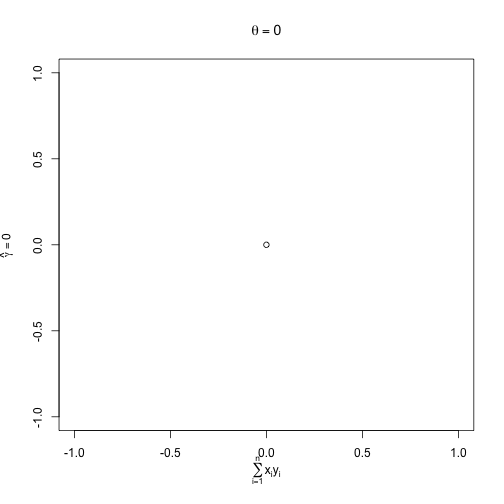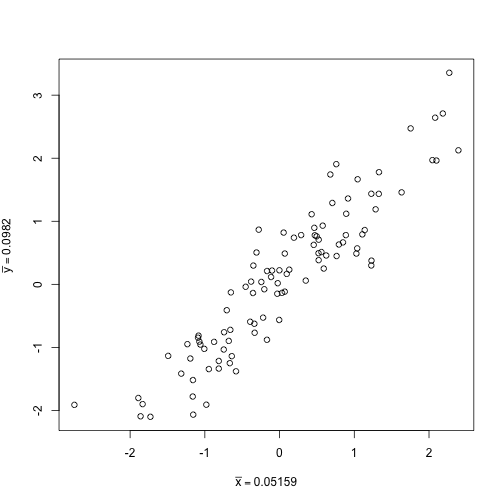Mathematical expressions can be included in the plot title or axis labels. For more information, see ?plotmath or run the demo with demo(plotmath).

Here are some quick examples.

Example 1

plot(0, 0, main = expression(theta == 0), ylab = expression(hat(gamma) == 0),
xlab = expression(sum(x[i] * y[i], i == 1, n)))Example 2

Strings can be pasted together with mathematic expressions:

x <- rnorm(100)
hist(x, xlab = expression("The mean (" * bar(x) * ") is " * sum(x[i]/n, i ==
1, n)))Example 3

The subsitute function can be used to substitute values into a mathematical expression.

x <- rnorm(100)
y <- x + rnorm(100, sd = 0.5)
plot(x, y, xlab = substitute(bar(x) == k, list(k = mean(x))), ylab = substitute(bar(y) ==
k, list(k = mean(y))))Go Top